Question

2. A normal dose of ibuprofen is 200. mg. How many ibuprofen molecules are there in a dose of ibuprofen? The molecular formula of ibuprofen is C13H18O2. (Hint: grams to mol and mol to molecules)

3-Propane fuel is used in BBQs. Use the following reaction to determine how many moles of carbon dioxide gas are produced from burning of 6 moles of propane gas.
C3H8(g) + 5O2(g) ®  4H2O(g) + 3CO2(g)

4- A. If a person inhales 51 moles of oxygen, how many grams of oxygen molecules are being inhaled?

B. How many molecules are being inhaled?

2.
The molecular formula of ibuprofen is C13H18O2
The atomic masses of C, H and O are 12 g/mol, 1 g/mol and 16 g/mol respectively.
The molar mass of ibuprofen is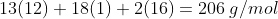200 mg = 0.200 g
Number of moles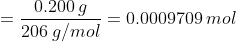Number of molecules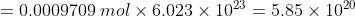molecules

3.
The burning of 1 mole propane produces 3 moles of CO2.
The burning of 6 mole propane produces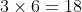moles of CO2

4.A
The molar mass of oxygen is 32 g/mol.
Grams of oxygen molecules inhaled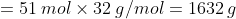B
Number of molecules being inhaled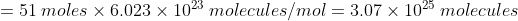#### Earn Coins

Coins can be redeemed for fabulous gifts.

Similar Homework Help Questions
• ### How molecules of CO2 (g) are produced when 0.75 moles of propane are burned? Chemical reaction:...

How molecules of CO2 (g) are produced when 0.75 moles of propane are burned? Chemical reaction: C3H8 + 5O2 à 3CO2 + 4H2O

• ### Ibuprofen is an anti-inflammatory with the formula C13H18O2. How many grams of ibuprofen are in 0.495...

Ibuprofen is an anti-inflammatory with the formula C13H18O2. How many grams of ibuprofen are in 0.495 mol? How many moles of carbon are in 10.0 g of ibuprofen? How many moles of ibuprofen contain 1.15×1023 atoms of carbon?

• ### ChemistryConsider the combustion of propane gas,C3H8(g) + 5O2(g) 3CO2(g) +4H2O(l)Ho = -2,220 kJ/molPropane (just C3H8) is often used for gasgrills. Anyone who has every filled or moved those tanks knows theycan get pretty heavy.a) How many grams of propane are in 1 pounds ofpropane? Use the conversion 1 lb = 454 g. (Express your answersfor the next three questions in scientificnotation. For exampleuse 2.3e-5 to indicate a number such as 2.3 x10-5.)gramsb) How many moles of propane are in 1...

• ### I am still not quite grasping how to complete this problem: Posted by K on Sunday, October 14, 2007 at 6:43pm

I am still not quite grasping how to complete this problem:Posted by K on Sunday, October 14, 2007 at 6:43pm. Many home barbeques are fueled with propane gas C3H8. What mass of carbon dioxide (in kg) is produced upon the complete combustion of 18.9L of propane (approximate contents of one 5-gallon tank)? Assume that the density of the liquid propane in the tank is 0.621g/ml. How do I start & work this question? I wrote a balanced equation: C3H8 +...

• ### 1. Will Cd + Zn2+ react? 2. Is Ni(OH)2 soluble in H2O? 3. Propane burns according...

1. Will Cd + Zn2+ react? 2. Is Ni(OH)2 soluble in H2O? 3. Propane burns according to the following equation:                  C3H8 + 5O2 3CO2 + 4H2O      How many grams of CO2 can form from 0.177 mol of propane? 4. Cinnamic acid is 72.96% carbon, 5.40% hydrogen, and the rest is oxygen. What is the empirical formula of this acid?

• ### Chemistry ch9Considerthe combustion of propane gas,C3H8(g) +5O2(g) 3CO2(g)+ 4H2O(l)Ho =-2,220 kJ/molPropaneis often used for gas grills and is typically purchased by fillinga propane tank with 20 pounds of propane. How much heat canbeobtained by burning 8 pound of propane? Use the conversion 1 lb =454 g and express your answer in scientific notation. For exampleuse 2.3e-5 to indicate a numbersuch as 2.3 x10-5.

• ### Many home barbeques are fueled with propane gas C3H8

Many home barbeques are fueled with propane gas C3H8.What mass of carbon dioxide (in kg) is produced upon the complete combustion of 18.9L  of propane (approximate contents of one 5-gallon tank)? Assume that the density of the liquid propane in the tank is 0.621g/ml.How do I start & work this question?I wrote a balanced equation:C3H8 + 5O2 -> 4H2O + 3CO2Now, where do I go from here?

• ### How many grams of O2(g) are needed to completely burn 35.9 g of C3H8(g)? C3H8 +...

How many grams of O2(g) are needed to completely burn 35.9 g of C3H8(g)? C3H8 + 5O2 ---> 3CO2 + 4H2O

• ### If we have C3H8 + 5O2 ---> 3CO2 + 4H2O a) How many liters of oxygen...

If we have C3H8 + 5O2 ---> 3CO2 + 4H2O a) How many liters of oxygen gas are required to react with 7.2 L of C3H8 if both gasses are at STP? b) How many grams of CO2 will be produced from 35 L C3H8 at 15 C and 1.65 atm? c) How many grams of water vapor can be produced when 15 L C3H8 at 15 C and 1.65 atm are reacted with 15 L O2 at -20.0 C...

• ### For the gas propane (C3H8), (a) how many grams are in 1.50 moles of C3H8, (b)...

For the gas propane (C3H8), (a) how many grams are in 1.50 moles of C3H8, (b) how many moles are in 25.0 grams of C3H8, (c) how many molecules of C3H8 are in 25.0 moles of C3H8 and finally (d) what is the percent carbon in C3H8?